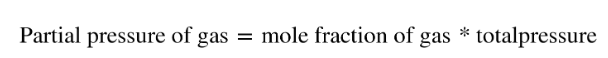# Problem: A gas mixture contains 75.2% nitrogen and 24.8% krypton by mass.What is the partial pressure of krypton in the mixture if the total pressure is 776 mm Hg ?

###### FREE Expert Solution
• Convert this following mass percent to moles to find the mole fraction of N2 and Kr.
• Then use provided total pressure to determine the partial pressure of each gas by multiplying the mole fraction of gas to the total P of solution:• Assume that the % mass given were mass in grams, then convert it to moles

MM of N2: N - 14.01 (2) = 28.02 g/mol

MM of Kr: 83.80 g/mol

92% (474 ratings)###### Problem Details

A gas mixture contains 75.2% nitrogen and 24.8% krypton by mass.

What is the partial pressure of krypton in the mixture if the total pressure is 776 mm Hg ?